Open in App
Not now

# Scraping Wikipedia table with Pandas using read_html()

• Last Updated : 02 Aug, 2022

In this article, we will discuss a particular function named read_html() which is used to read HTML tables directly from a webpage into a Pandas DataFrame without knowing how to scrape a website’s HTML, this tool can be useful for swiftly combining tables from numerous websites. However, the data must have to be cleaned further, So let’s see how we can work on these data.

Pandas read_html() is one of the easiest ways to scrape web data. The data can further be cleaned as per the requirements of the user.

```Syntax: pandas.read_htlm(io)
Where, io can be an HTML String, a File, or a URL.```

### Example 1: Using an Html string

In this example, we are storing a multiline string using the notation ‘’’ in a variable called html_string. Then, we call the function read_html and pass the html_string to it. This function extracts all the HTML tables and returns a list of all the tables.

## Python3

 `import` `pandas as pd ` ` `  `html_string ``=` `''' ` `  `` ` `  ``
CompanyContactCountry
Alfreds FutterkisteMaria AndersGermany
Centro comercial MoctezumaFrancisco ChangMexico
` `    `` ` `    `` ` `    `` ` `  `` ` `  `` ` `    `` ` `    `` ` `    `` ` `  `` ` `  `` ` `    `` ` `    `` ` `    `` ` `  `` ` ` ` `'''` `df_1 ``=` `pd.read_html(html_string) ` `df_1 `

Output: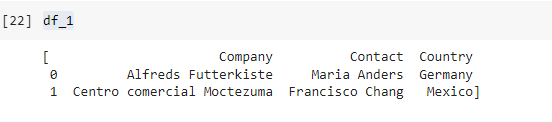Further, if you want to look at the datatypes, you can do so by calling the info() function as follows:

`df_1.info()`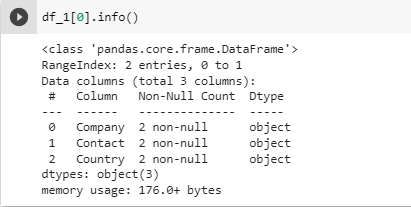### Example 2: Reading HTML Data From URL

In this example, let us try to read HTML from a web page. We are using a Wikipedia page with the url=” Demographics_of_India”. From this webpage, I want to scrape the contents of the following table, We need to extract the highlighted columns below: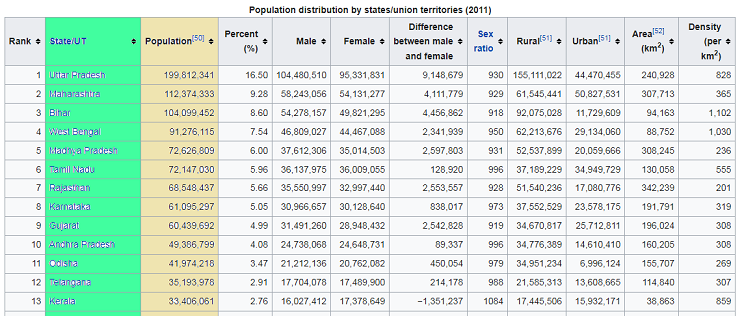There are almost 37 tables on the webpage and to find a particular table, we can use the parameter “match”. To find out the length of the data frame, we use the len() function as follows:

## Python3

 `import` `pandas as pd ` `import` `numpy as np ` ` `  `dfs ``=` `pd.read_html('https:``/``/``en.wikipedia.org\ ` `/``wiki``/``Demographics_of_India') ` `len``(dfs) `

Output:

`37`

### Example 3: Find the specific table from a webpage

Let us pass the value “Population distribution by states/union territories (2011)” to the parameter match.

## Python3

 `my_table ``=` `pd.read_html('https:``/``/``en.wikipedia.org``/``wiki``/``\ ` `Demographics_of_India', ` ` ``match``=``'Population distribution by states/union territories'``) ` `my_table[``0``].head() `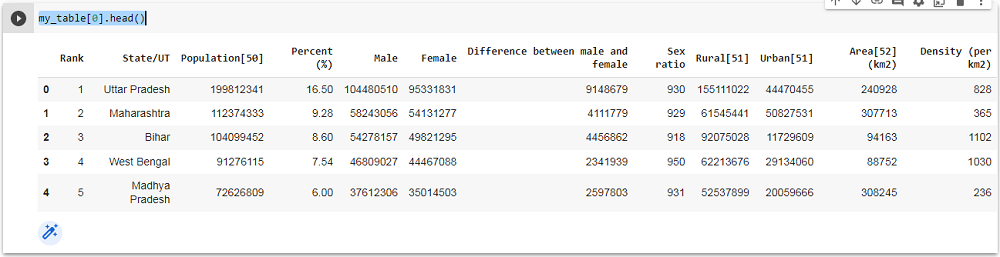### Example 4: Fetch column data

So, we have to get the column ‘State/UT’ and also the column ‘Population’

## Python3

 `states ``=` `my_table[``0``][``'State/UT'``] ` `states `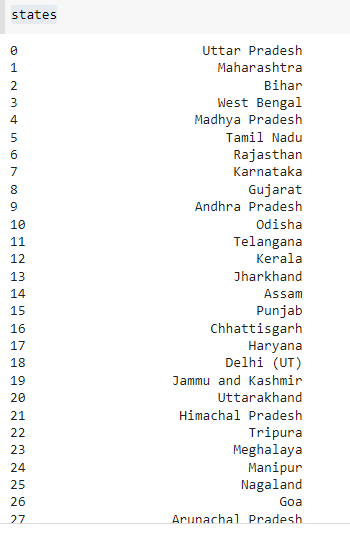Similarly, we get the column Population

## Python3

 `population ``=` `my_table[``0``][``'Population'``] ` `population`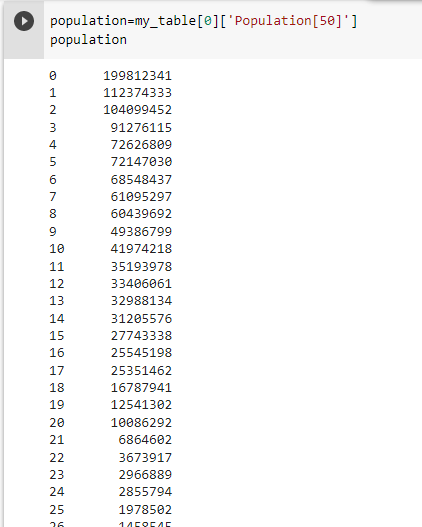### Example 5: Merging two columns

Let us store the two columns in a new DataFrame.

## Python3

 `df1 ``=` `pd.DataFrame({``'State'``: states,  ` `                    ``'Population'``: population}) ` `df1 `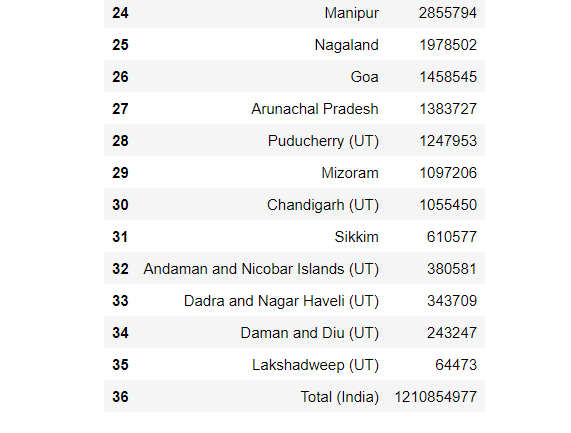### Example 6: Dropping row  data

Let’s try to Remove the last row with the help of drop() in Pandas. i.e. total

## Python3

 `df1.drop(df1.tail(``1``).index, ` `        ``inplace ``=` `True``) ` `df1`

Output: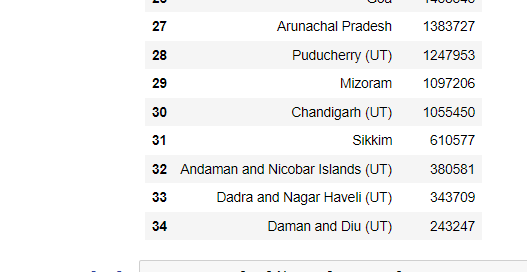### Example 7: Data Visualisation of table

Here we are using the Matplotlib module to plot the given HTML data in a graphic format.

## Python3

 `import` `matplotlib.pyplot as plt ` ` `  `df1.plot(x``=``'State'``,y``=``'Population'``, ` `         ``kind``=``"barh"``,figsize``=``(``10``,``8``))`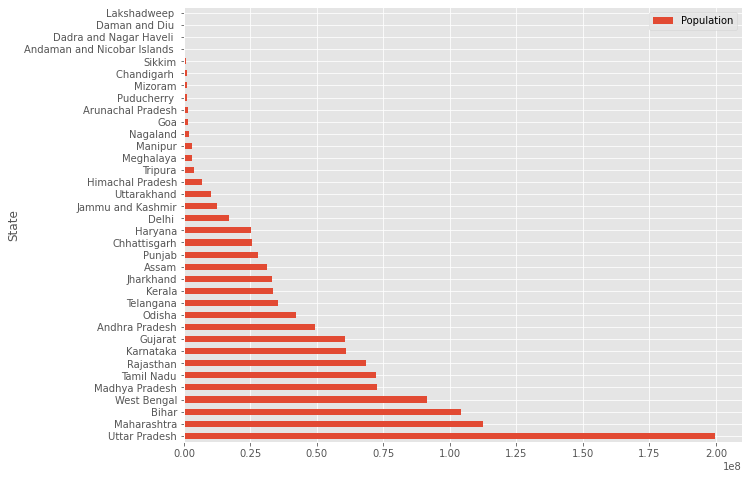### Example 8: Writing HTML Tables with Python’s Pandas

Here, we created a DataFrame, and we converted it into an HTML file, we have also passed some HTML attributes for making it table beautiful.

## Python3

 `import` `pandas as pd ` ` `  `df ``=` `pd.DataFrame({``'A'``: [``1``, ``2``], ``'B'``: [``3``, ``4``]}) ` `df.to_html(``'write_html.html'``, index``=``False``,  ` `           ``border``=``3``, justify``=``'center'``)`

Output: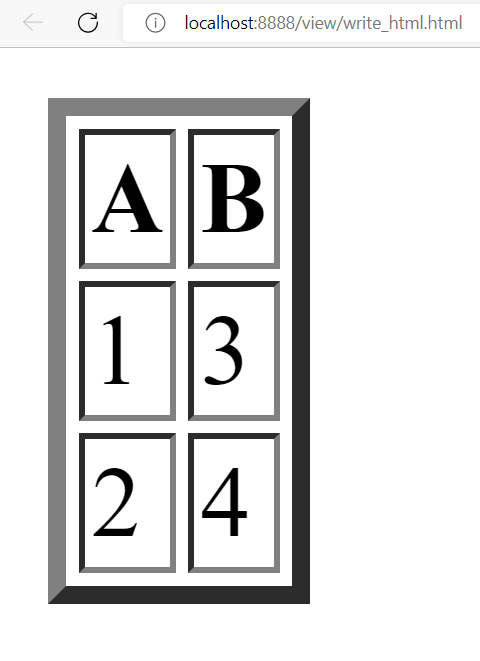### Example 9: Error while rendering an HTML page

If the HTML page doesn’t contain any tables, a value error will return.

## Python3

 `import` `pandas as pd ` `import` `numpy as np ` ` `  `dfs``=``pd.read_html('https:``/``/``codebestway.\ ` `wordpress.com``/``')`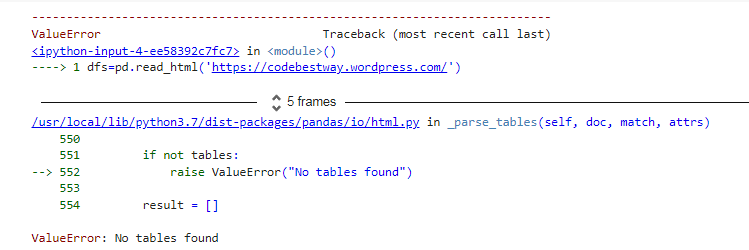My Personal Notes arrow_drop_up
Related Articles